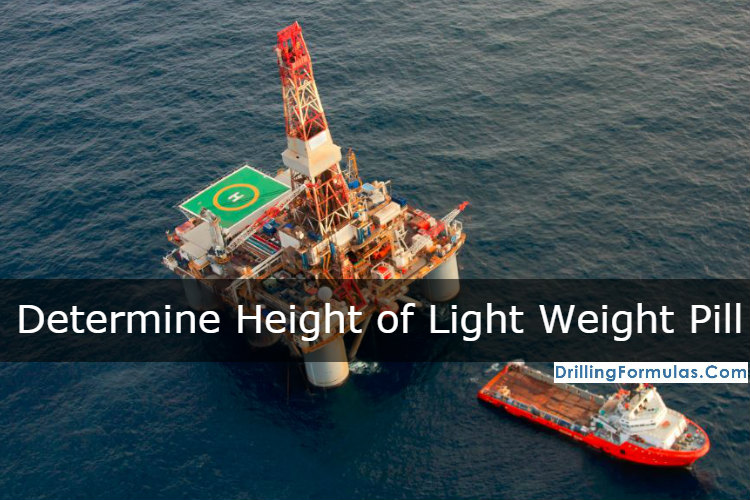# Determine height of light weight spot pill to balance formation pressure

When you get differentially stuck, you may consider spotting light weight fluid to reduce force created by differential pressure between mud in wellbore and formation pressure. However, it is imperative to be able to calculate how much light weight fluid added into the well that it will not  accidentally underbalance formation pressure.Please follow the steps below to determine how height of light weight spot pill in the annulus to balance formation pressure.

a) Determine the difference in pressure gradient in psi/ft between the mud weight and light weight spot fluid:

Difference pressure in pressure gradient in psi/ft = (current mud wt in ppg – light weight spot pill in ppg) x 0.052

b) Determine height in ft of light weight spot fluid that will balance formation pressure in the annulus:

Height ft in vertical = overbalance pressure with current mud weigh in psi ÷ difference in pressure gradient in psi/ft

This height is the maximum allowable height of light weight spot pill in the annulus. If you have higher length of light weight spot pill in the annulus, it may create a well control situation.

Example: Use the following data to determine the height in ft of light weight spot pill that will balance formation pressure in the annulus:

Mud weight = 13.0 ppg

Light weight spot pill = 8.3 ppg

Amount of overbalance = 300 psi

a) Difference in pressure gradient in psi/ft:

Difference pressure in pressure gradient in psi/ft = (13 ppg – 8.3 ppg) x 0.052

Difference pressure in pressure gradient = 0.2444 psi/ft

b) Determine the height in ft of light weight spot liquid that will balance formation pressure in the annulus:

Height = 300 psi ÷ 0.244 psi/ft

Height = 1227 ft

You must ensure that height of light weight pill in the annulus must be less than 1227 ft in order to prevent a wellcontrol situation.

In order to determine light weight pill volume, this simple formula below is used;

volume of pill = annular capacity (bbl/ft) x height of pill (ft)

For instant, the annular capacity is 0.0459 bbl/ft, volume of pill is equal to 56.3 bbl (1,227 x 0.0459).

Ref books: Lapeyrouse, N.J., 2002. Formulas and calculations for drilling, production and workover, Boston: Gulf Professional publishing.

Bourgoyne, A.J.T., Chenevert , M.E. & Millheim, K.K., 1986. SPE Textbook Series, Volume 2: Applied Drilling Engineering, Society of Petroleum Engineers.

Mitchell, R.F., Miska, S. & Aadny, B.S., 2011. Fundamentals of drilling engineering, Richardson, TX: Society of Petroleum Engineers.

Share the joy